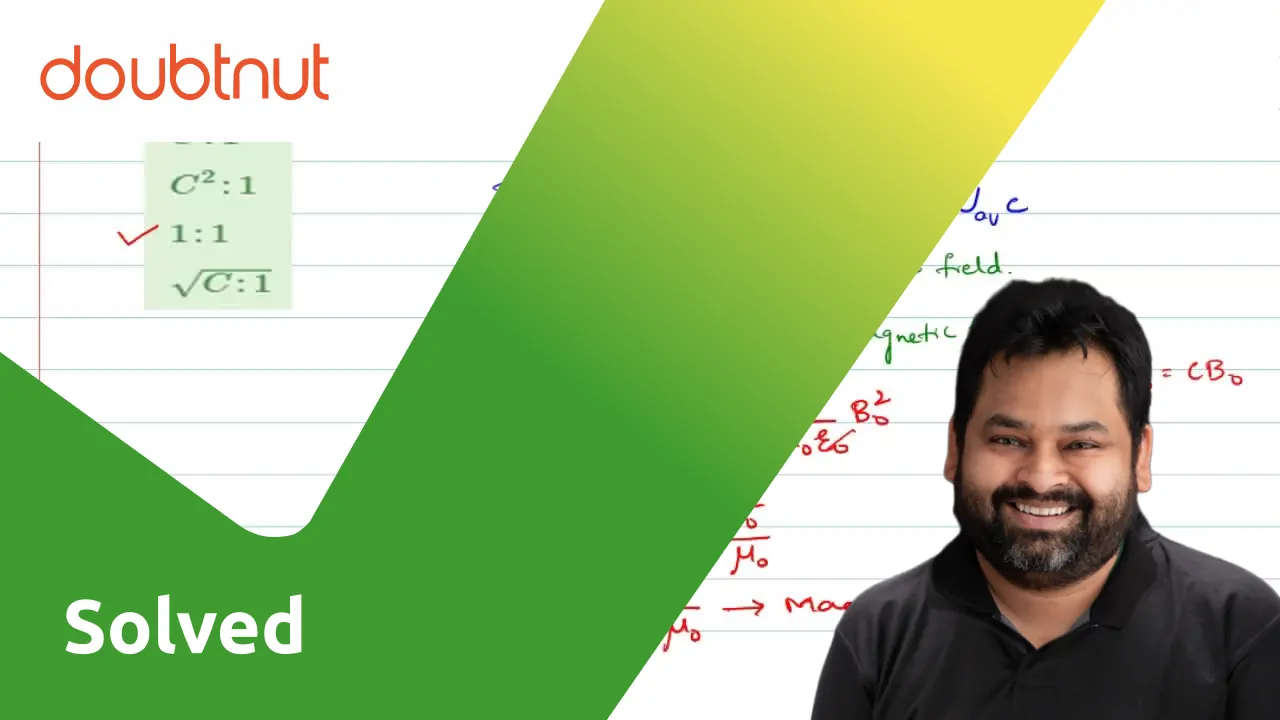Doubtnut App - Get 50 Lakh+ video solutions better than GoogleInstall Now

# The ratio of contributions made by the eletric field and magnetic field components to the intensity of an EM wave is.

A

C:1

B

C2:1

C

1:1

D

C:1

Text Solution
Verified by Experts

## Intensity in terms of electric field Uav=12ε0E20 Intensity in terms of magnetic field Uav=12B20μ0 Now taking the intensity in terms of electric field. (Uav) electric field =12ε0E20 ⇒ =12ε0(cB0)2 (E0=cB0) =12ε0×c2B2 , But c=1√μ0ε0 ∴ (Uav)Electric field=12ε0×1μ0ε0B20=12B20μ0 =(Uav)magnetic field Thus the energy in electromagnetic wave is divided equally between electric field vector and magnetic field vector. Therefore the of contributions by the electric field and magnetic field components to the intensity of an electromagnetic wave is 1:1